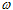# Block Reference

The Blocks menu and Menu Browser list the standard blocks provided with Embed. You can also access blocks from the Blocks toolbars. For most blocks, the corresponding mathematical function is included. The following table explains the symbols that may appear in the function:

 Symbol What it represents A Amplitude e Napierian constant dt Derivative with respect to time lb Lower bound mod Modulus s Laplacian operator t Time ub Upper boundFrequency x Input signal y Output signal

Input signals are represented as x1, x2, …xn where x1 represents the topmost signal entering the block. When n is omitted, x1 is assumed. Output signals are represented as y1, y2,…yn, where y1 represents the topmost signal exiting the block. When n is omitted, y1 is assumed.